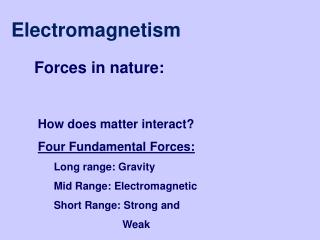DownloadDownload PresentationElectromagnetism

# Electromagnetism

Download Presentation## Electromagnetism

- - - - - - - - - - - - - - - - - - - - - - - - - - - E N D - - - - - - - - - - - - - - - - - - - - - - - - - - -
##### Presentation Transcript

1. Electromagnetism Forces in nature: • How does matter interact? • Four Fundamental Forces: • Long range: Gravity • Mid Range: Electromagnetic • Short Range: Strong and • Weak

2. Electric Charge (~Mass) Two types of electric charge: positive and negative Unit of charge is the coulomb [C] Charge of electron (negative) or proton (positive)is Charge is quantized Charge is conserved

3. Electric Force (~Gravity) • The electric force between charges q1 and q2 is • repulsive if charges have same signs • (b) attractive if charges have opposite signs Like charges repel and opposites attract !!

4. Coulomb's Law Coulomb’s law: Force by q1 on q2 Coulomb’s constant in vacuum: Direction: along the line joining the two charges Repulsive or attractive

5. The superposition principle If many charges are present: Net force is on any charge is the vector sum of the forces from all the individual charges In general:

6. The electric field The electric field at a point is the force acting on a test charge q at that point divided by the charge q For a test charge q has the direction and sense of the force on a positive charge.

7. Electric field created by a point charge Q is outwards a positive charge and inwards a negative charce

8. The superposition principle for fields The electric field due to a collection of N point charges is the vector sum of the individual electric fields due to each charge:

9. Example of vector field: gravitation

10. Comparison with gravity

11. Electric field lines

12. Flows With Sources (http://ocw.mit.edu/ans7870/8/8.02T/f04/visualizations/vectorfields/02-particleSource/02-ParticleSource_320.html)

13. Flows with sinks (http://ocw.mit.edu/ans7870/8/8.02T/f04/visualizations/vectorfields/01-particleSink/01-ParticleSink_320.html)

14. Circulating flows (http://ocw.mit.edu/ans7870/8/8.02T/f04/visualizations/vectorfields/03-particleCirculate/03-PartCircMotion_320.html)